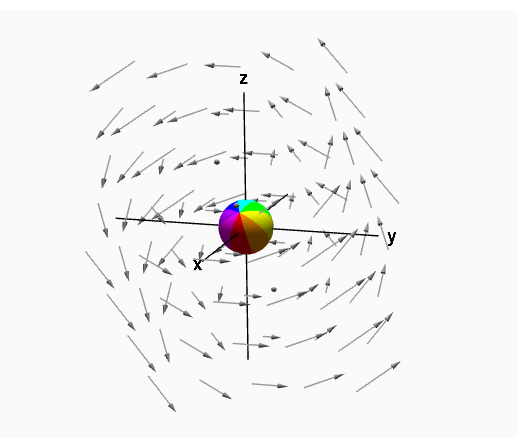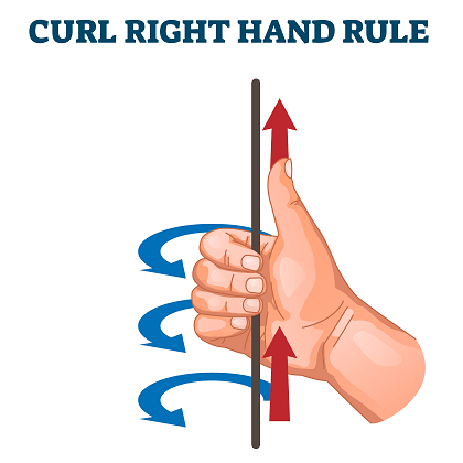Uh Oh! It seems you’re using an Ad blocker!

Since we’ve struggled a lot to makes online calculations for you, we are appealing to you to grant us by disabling the Ad blocker for this domain.# Curl Calculator

$$\mathbf{\vec{F}}\left(x,y,z\right)$$:

x:

y:

z:

$$\left(x_{0}, y_{0}, z_{0}\right)$$: (optional)

$$x_{0}$$:

$$y_{0}$$:

$$z_{0}$$:

Table of Content

 1 What is curl calculus? 2 Formula of curl: 3 How to find the curl of a vector field? 4 What does curl 0 mean? 5 Is the curl positive or negative? 6 What is meant by divergence of a vector? 7 Where curl is used?

Get the Widget!

Add this calculator to your site and lets users to perform easy calculations.

Feedback

How easy was it to use our calculator? Did you face any problem, tell us!

An online curl calculator is specially designed to calculate the curl of any vector field rotating about a point in an area.

If you are interested in understanding the concept of curl, continue to read.

## What is Curl Calculus?

In calculus, a curl of any vector field A is defined as:

The measure of rotation (angular velocity) at a given point in the vector field.The curl of a vector field is a vector quantity.

Magnitude of curl:

The magnitude of a curl represents the maximum net rotations of the vector field A as the area tends to zero.

Direction of the curl:

The direction of a curl is given by the Right-Hand Rule which states that:

“Curl the fingers of your right hand in the direction of rotation, and stick out your thumb. The vector representing this three-dimensional rotation is, by definition, oriented in the direction of your thumb.”With the help of a free curl calculator, you can work for the curl of any vector field under study.

### Formula of Curl:

Suppose we have the following function:

F = P i + Q j + R k

The curl for the above vector is defined by:

Curl = ∇ * F

First we need to define the del operator as follows:

$$\ ∇ = \frac{\partial}{\partial x} * {\vec{i}} + \frac{\partial}{\partial y} * {\vec{y}}+ \frac{\partial}{\partial z} * {\vec{k}}$$

So we have the curl of a vector field as follows:

$$\operatorname{curl} F= \left|\begin{array}{ccc}\mathbf{\vec{i}} & \mathbf{\vec{j}} & \mathbf{\vec{k}}\\ \frac{\partial}{\partial x} & \frac{\partial}{\partial y} & \frac{\partial}{\partial z}\\P & Q & R\end{array}\right|$$

Thus, $$\operatorname{curl}F= \left(\frac{\partial}{\partial y} \left(R\right) – \frac{\partial}{\partial z} \left(Q\right), \frac{\partial}{\partial z} \left(P\right) – \frac{\partial}{\partial x} \left(R\right), \frac{\partial}{\partial x} \left(Q\right) – \frac{\partial}{\partial y} \left(P\right) \right)$$

The same procedure is performed by our free online curl calculator to evaluate the results.

Rotational Vector:

A rotational vector is the one whose curl can never be zero.

For example:

Spinning motion of an object, angular velocity, angular momentum etc.

Irrotational Vector:

A vector with a zero curl value is termed an irrotational vector.

Curl = ∇ * F = 0

For example:

A fluid in a state of rest, a swing at rest etc.

### How To Find the Curl of a Vector Field?

To understand the concept of curl in more depth, let us consider the following example:

How to find curl of the function given below?

F = (cos(x), sin(xyz), 6x + 4)

Solution:

The given function is:

F = (cos(x), sin(xyz), 6x + 4)

As we know that, the curl is given by the following formula:

Curl = ∇ * F = 0

So, we have;

By definition, $$\operatorname{curl}{\left(\cos{\left(x \right)}, \sin{\left(xyz\right)}, 6x+4\right)} = \nabla\times\left(\cos{\left(x \right)}, \sin{\left(xyz\right)}, 6x+4\right)$$,

Or equivalently
$$\operatorname{curl}{\left(\cos{\left(x \right)}, \sin{\left(xyz\right)}, 6x+4\right)} = \left|\begin{array}{ccc}\mathbf{\vec{i}} & \mathbf{\vec{j}} & \mathbf{\vec{k}}\\\frac{\partial}{\partial x} &\frac{\partial}{\partial y} & \ {\partial}{\partial z}\\\\cos{\left(x \right)} & \sin{\left(xyz\right)} & 6x+4\end{array}\right|$$

$$\operatorname{curl}{\left(\cos{\left(x \right)}, \sin{\left(xyz\right)}, 6x+4\right)} = \left(\frac{\partial}{\partial y} \left(6x+4\right) – \frac{\partial}{\partial z} \left(\sin{\left(xyz\right)}\right), \frac{\partial}{\partial z} \left(\cos{\left(x \right)}\right) – \frac{\partial}{\partial x} \left(6x+4\right), \frac{\partial}{\partial x}\left(\sin{\left(xyz\right)}\right) – \frac{\partial}{\partial y}\left(\cos{\left(x \right)}\right) \right)$$

After evaluating the partial derivatives, the curl of the vector is given as follows:

$$\left(-x y \cos{\left(x \right)}, -6, \cos{\left(x \right)}\right)$$

You can also determine the curl by subjecting to free online curl of a vector calculator.

### How Online Curl Calculator Works?

The net rotational movement of a vector field about a point can be determined easily with the help of curl of vector field calculator. We need to know what to do:

Input:

• Put the values of x, y and z coordinates of the vector field

Now, if you wish to determine curl for some specific values of coordinates:

• Select the desired value against each coordinate
• Click ‘calculate’

Output:

With help of input values given, the vector curl calculator calculates:

• Curl of the vector field
• Step by step calculations to clarify the concept.

## FAQ’s:

### What does curl 0 mean?

As you know that curl represents the rotational or irrotational character of the vector field, so a 0 curl means that there is no any rotational motion in the field.

### Is the curl positive or negative?

A positive curl is always taken counter clockwise while it is negative for anti-clockwise direction. You can assign your function parameters to vector field curl calculator to find the curl of the given vector.

### What is meant by divergence of a vector?

The divergence of a vector is a scalar quantity that measures how a fluid collects or disperses at a particular point.

### Where curl is used?

Curl has a broad use in vector calculus to determine the circulation of the field. Using curl of a vector field calculator is a handy approach for mathematicians that helps you in understanding how to find curl.

## Conclusion:

Curl provides you with the angular spin of a body about a point having some specific direction. Curl has a wide range of applications in the field of electromagnetism. Apart from the complex calculations, a free online curl calculator helps you to calculate the curl of a vector field instantly.

From the source of Wikipedia: Intuitive interpretation, Descriptive examples, Differential forms, Curl geometrically

From the source of lumen learning: Vector Fields, Conservative Vector Fields, Path Independence, Line Integrals, Fundamental Theorem for Line Integrals, Green’s Theorem, Curl and Divergence, Parametric Surfaces and Surface Integrals, Surface Integrals of Vector Fields.

From the source of khan academy: Divergence, Interpretation of divergence, Sources and sinks, Divergence in higher dimensions